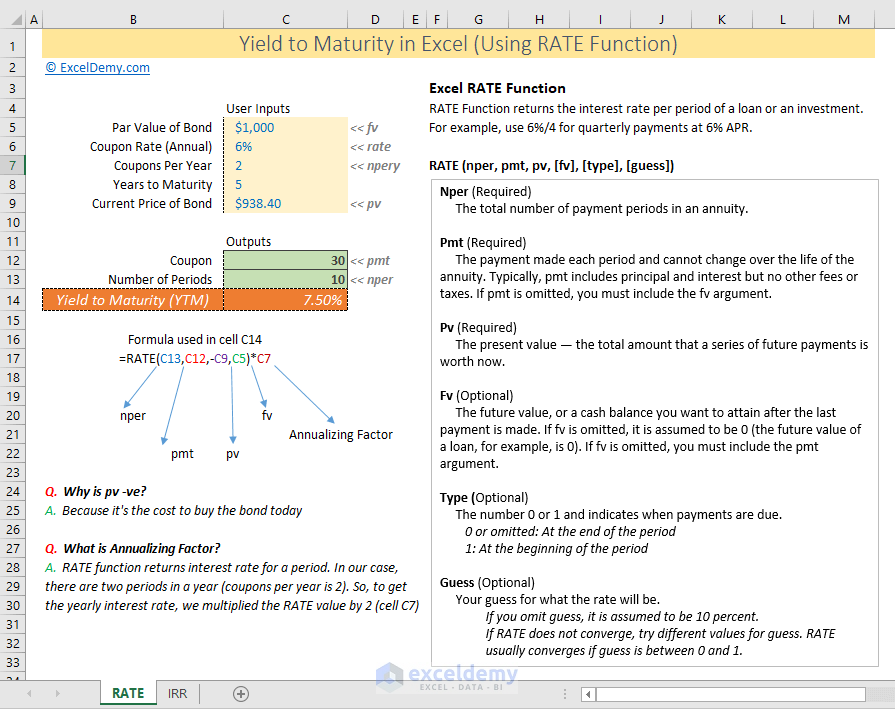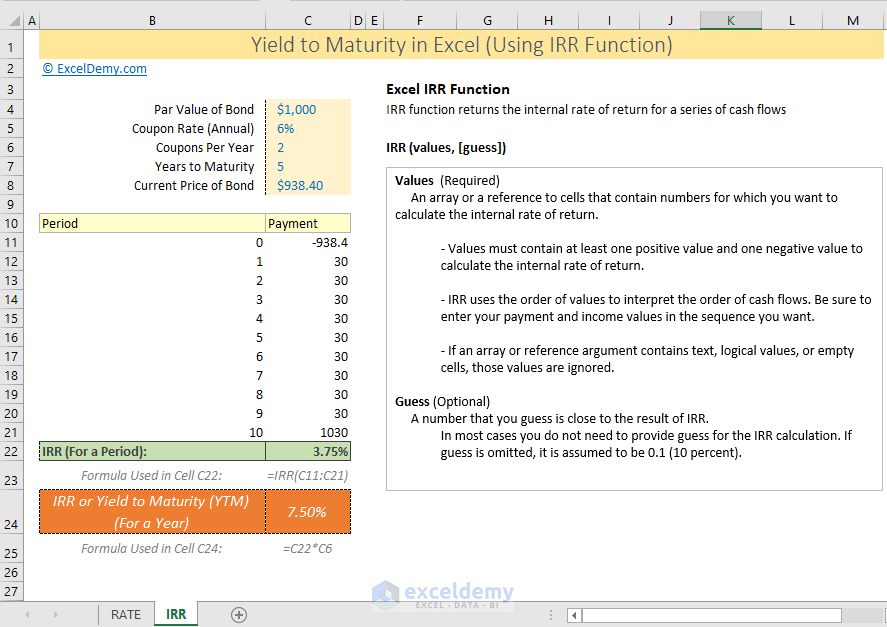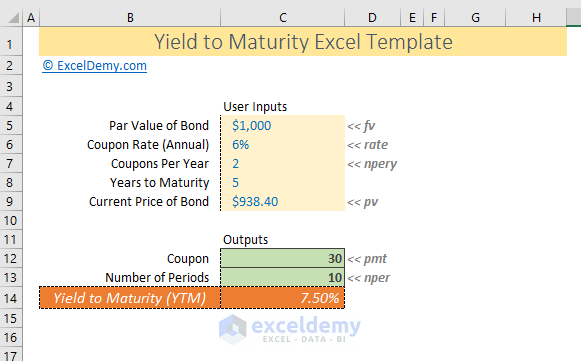Disclosure: This post may contain affiliate links, meaning when you click the links and make a purchase, we receive a commission.

# How to calculate yield to maturity in Excel (with Template)In this article, I will show how to calculate yield to maturity (YTM) in Excel. YTM is also known as the internal rate of return. I will calculate YTM in two ways:

• Using Excel’s RATE function
• Using Excel’s IRR function

## How to Calculate Yield to Maturity (YTM) in Excel

### 1) Using the RATE Function

Suppose, you got an offer to invest in a bond.

Here are the details of the bond:

• Par Value of Bond (Face Value, fv): \$1000
• Coupon Rate (Annual): 6%
• Coupons Per Year (npery): 2. The company pays interest two times a year (semi-annually).
• Years to Maturity: 5 years. From the time you buy the bond.
• Current Price of Bond (Present Value, pv): \$938.40

You’re wondering whether you would invest in the bond. To make this decision, you want to know the Yield to Maturity (also called Internal Rate of Return) from investing in the bond.

You can use Excel’s RATE function to calculate the Yield to Maturity (YTM). Check out the image below.The syntax of RATE function:

RATE (nper, pmt, pv, [fv], [type], [guess])

Here,

Nper = Total number of periods of the bond maturity. Years to maturity of the bond is 5 years. But coupons per year is 2. So, nper is 5 x 2 = 10

Pmt = The payment made in every period. It cannot change over the life of the bond. The coupon rate is 6%. But as payment is done twice a year, the coupon rate for a period will be 6%/2 = 3%. So, pmt will be \$1000 x 3% = \$30.

PV = Present value of the bond. It is the amount that you spend to buy a bond. So, it is negative in the RATE function.

FV = Future value of the bond. It is actually the face value of the bond. When the bond matures, you get return the face value of the bond. In our case, it is \$1,000.

Type = Type can be either 0 or 1 or omitted. If 0 or omitted, the interest payment (coupon payment or pmt) is done at the end of the period. If the type is 1, the coupon payment is done at the beginning of the period.

Guess = It is just a guess value. I did not use it. In most cases (if not all cases), don’t use this value.

I also have used another term in the formula. It is an Annualizing Factor.

What is the Annualizing Factor?

RATE function returns the interest rate for a period. In our case, there are two periods per year (coupons per year is 2). So, to get the yearly interest rate, we multiplied the RATE value by 2 (cell C7).

### 2) Using Excel’s IRR Function

This is an easy and straightforward way of calculating YTM in Excel.You see I have just entered the future cash flows from the bond investments in a column (Payment column) and then used Excel’s IRR function. The formula gives us the internal rate of return for a period: 3.75%

Later, I have multiplied this value (3.75%) by 2 as the bond pays two times (semi-annually) a year.

The syntax of the IRR function:

IRR (values, [guess])

Here,

Values = The future cash flows of the bonds. The values must contain a positive value and a negative value. The bond cost \$938.40, so it is a negative value at the start of the ‘Payment’ column. And at the end of the bond maturity, we get the coupon payment and the face value back, so it is \$1030. Between these two, we get \$30 in every period. These are the cash flows for the next 5 years (10 periods).

Guess = It is just a guess value that could be your internal rate of return. I did not use this value.

The IRR function returns the internal rate of return for a period. This is why we have multiplied this return by 2 to get the yearly internal rate of return.

That’s all.

If you want to know other ways of calculating the internal rate of return, check this article: How to calculate IRR (internal rate of return) in Excel (9 easy ways)

## Yield to Maturity (YTM) Excel Template

Use this Excel template to calculate the Yield to Maturity (YTM) in Excel.Input required values in the ‘User Inputs’ section and you will get the YTM automatically (lower part of the template). So easy to use and straightforward.

## Why is a Bond Sold in Discount or Premium?

A bond might sell in both discounts or premiums. It depends on the market rate of similar bonds.

### Why Bonds are Sold in Discount?

Suppose, you’re a company and you need some money to run your business.

You have made a plan to issue a bond with the following details:

• Face Value, fv = \$100,000
• Coupon Rate, rate = 6%
• Coupons per Year, npery = 4 (quarterly)
• Years of Maturity = 10

Now, you went to a bond rating agency (Moody’s, S&P, Fitch, etc.) and they rated your bond as AA+. More about the bond rating.

But the problem is: when you tried to sell the bond, you see that the same rated bond is selling with 7.5% YTM (yield to maturity). So, it will happen that you will not be able to sell the bond at face value.

This is when you will sell your bond at a discount.

How will you set the price of a bond in a discount? Very simple. Use Excel’s PV function.

=-PV (rate, nper, pmt, [fv], [type])

=-PV (7.50%/4, 4*10, 1500, 100,000)

= 89,513.17

Here for the rate argument, I have used the value of 7.50% (also divided it by 4 to get the period interest). Because I want to discount the cash flows with the market rate.

Nper = Maturity Years x Number of payments a year

Pmt = Face Value x (Coupon Rate/4)

And Face Value, fv = \$100,000

And this formula gives us to value: \$89,513.17

Before the PV function, I have used -ve sign to make the Present Value positive. Present Value is -ve because it is the cost to buy the bond.

### Why Bonds are Sold in Premium?

The scenario can also be different for the above bond.

You went to sell your bond and found that same rated bonds are selling with the market rate (YTM) 4.5%.

In this case, you will not want to sell your bond at 6% YTM. You will want a higher price for your bond so that yield to maturity from your bond will be 4.5%.

Let’s calculate now your bond price with the same Excel PV function.

=-PV (4.50%/4, 4*10, 1500, 100,000)

= \$112,025.59

So, you will be able to sell your bond at \$112,025.59 with a premium of amount \$12,025.59.

## Conclusion

So, that is my two ways of calculating yield to maturity (YTM) in Excel.

To check more ways, you can check out this link: How to calculate IRR (internal rate of return) in Excel (9 easy ways). YTM and IRR actually the same thing. It is all about future cash flows and their present values discounted with an interest rate.

Hello!
Welcome to my Excel blog! It took me some time to be a fan of Excel. But now I am a die-hard fan of MS Excel. I learn new ways of doing things with Excel and share here. Not only how to guide on Excel, but you will get also topics on Finance, Statistics, Data Analysis, and BI. Stay tuned!

1. ReplyAug 21, 2019 at 4:58 PM

This is a great work, clear and easy to understand.
Thanks

2. ReplyOobosae Jan 14, 2020 at 8:28 PM

Great work. Thanks for sharing

• ReplyJan 15, 2020 at 4:41 PM

3. Reply